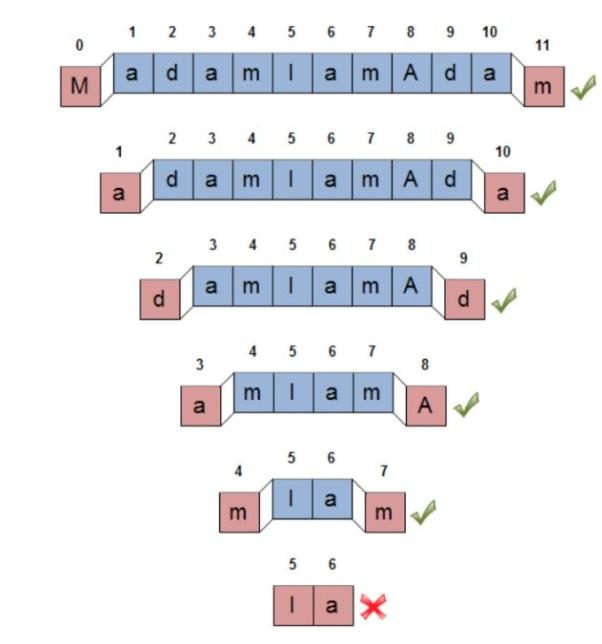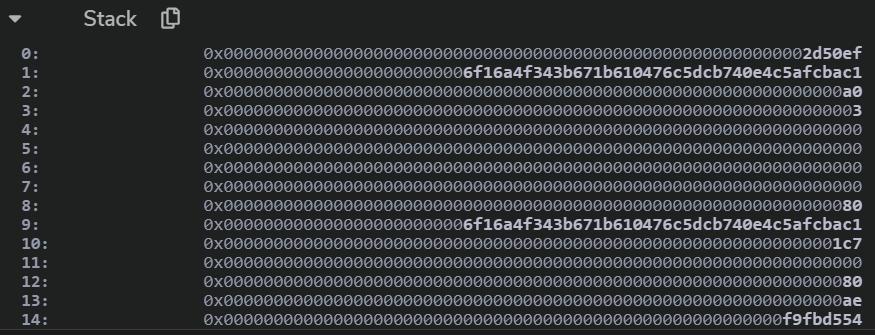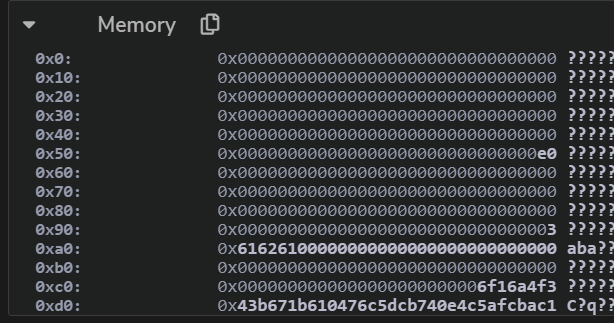## 题目分析## 测试用例：

``````testcases = {
"": True,
"a": True,
"ab": False,
"aba": True,
"tattarrattat": True,
}``````

## 思路：

### 参数判断 :muscle:

``````0xf9fbd554 => 函数选择器
0000000000000000000000000000000000000000000000000000000000000003 => tail 字节长度
6162610000000000000000000000000000000000000000000000000000000000 => tail "aba"的字节数组``````

\$\$ tail(X_i) = enc(X_i) \$\$

1. 先转换成字节数组 “aba” => 0x616261
2. 得到字节数组的长度 len(0x616261) = 0x03
3. 将字节数组左对齐 0x616261 =>0x6162610000000000000000000000000000000000000000000000000000000000

\$\mathtt{gas}=2d5cc5\$, \$\mathtt{to}=5c9eb5d6a6c2c1b3efc52255c0b356f116f6f66d\$, \$\mathtt{In offset}=C0\$, \$\mathtt{Insize}=04\$,\$\mathtt{Outoffset}=C0\$,\$\mathtt{OutSize}=20\$

``````calldata=> 我们发现calldata前面差4位，其是从[04:C4]
xxxxxxxx00000000000000000000000000000000000000000000000000000000
0000000000000000000000000000000000000000000000000000000000000000
00000000000000000000000000000000000000000000000000000000000000c0
0000000000000000000000000000000000000000000000000000000000000000
0000000000000000000000000000000000000000000000000000000000000003
6162610000000000000000000000000000000000000000000000000000000000
3ca7255b``````

\$\mathtt{gas}=2d50ef\$, \$\mathtt{to}=6f16a4f343b671b610476c5dcb740e4c5afcbac1\$, \$\mathtt{In offset}=a0\$, \$\mathtt{Insize}=03\$,\$\mathtt{Outoffset}=00\$,\$\mathtt{OutSize}=00\$ 此时的calldata是0x616261, 然后就进入了我们自定义的回文逻辑中。### 回文逻辑

``````{
//要比较的字符串在calldata中，其是一个只读的数据结构，需要首先拷贝到内存A中
calldatacopy(0x00, 0x00, calldatasize())
//初始化两个指针，left指向首位A，right指向末位(A+calldatasize()-1)
let left := 0x00
let right := sub(calldatasize(),1)
//比较指针left，right指向的值，如果不等就return false。如果相等，就left++, right--, 直到不满足left &lt; right的条件
for {} lt(left, right) {} {
if iszero(eq(left_r, right_r)) {
mstore(0x00, 0)
return(0x00, 0x20)
}
right := sub(right, 0x01)
}
//最后return true回去
mstore(0x00, 1)
return(0x00, 0x20)
}``````

``````{
let i := returndatasize()
let m := shr(selfbalance(), calldatasize())
let e := sub(calldatasize(), selfbalance())
for {} and(
lt(i, m)
) {i := add(i, selfbalance())} {}

mstore(callvalue(), eq(i, m))
return(callvalue(), 0x20)
}``````

1. 使用returndatasize()来代替 push1 0x00
2. 使用selfbalance()来代替 Push1 0x01
4. 使用 i == len(calldata)/2 来判定是否成功，如果相等则返回True，如果不等，则返回False。而不是上面写的分别return True和return False

``````{
//要比较的字符串在calldata中，其是一个只读的数据结构，需要首先拷贝到内存A中
//初始化两个指针，left指向首位A，right指向末位(A+calldatasize()-1)
let left := returndatasize()
let right := sub(calldatasize(),selfbalance())
//比较指针left，right指向的值，如果不等就return false。如果相等，就left++, right--, 直到不满足left &lt; right的条件
for {} lt(left, right) {} {
// 不匹配，return false 回去
if iszero(eq(left_r, right_r)) {
mstore(0x00, 0)
return(0x00, 0x20)
}
right := sub(right, 0x01)
}
//最后return true回去
mstore(0x00, 1)
return(0x00, 0x20)
}``````

## 节省GAS

### 提示4：通过重复使用变量的旧拷贝来避免POP

``````0010  DUP1              [i i size]
...
0020  DUP1              [i i size]
0021  SELFBALANCE       [1 i i size]
0023  SWAP1             [i i+1 size]
0024  POP               [i+1 size]``````

``````0010  DUP1              [i i size]
0011  SELFBALANCE       [1 i i size]
0013  SWAP1             [i i+1 size]
...``````

### 提示5：利用执行错误来恢复交易

``````pc    instruction       stack
----  --------------    ----------------------------------
0000  CALLDATASIZE      size
0001  RETURNDATASIZE    size 0

0002  JUMPDEST
0003  DUP1              size i i
0004  SELFBALANCE       size i i 1
0006  SWAP1             size i+1 i

0008  DUP2              size i+1 data[i] i+1
0009  DUP4              size i+1 data[i] i+1 size
000A  SUB               size i+1 data[i] size-i-1
000B  CALLDATALOAD      size i+1 data[i] data[size-i-1]
000C  XOR               size i+1 xor
000D  RETURNDATASIZE    size i+1 xor 0
000E  BYTE              size i+1 byte
000F  RETURNDATASIZE    size i+1 byte 0
0010  JUMPI             size i+1 // revert 如果byte != 0，则revert

0011  DUP2              size i+1 size
0012  DUP2              size i+1 size i+1
0013  LT                size i+1 cont
0014  PUSH1 0x02        size i+1 cont 0x02
0016  JUMPI             size i+1 //
0017  STOP              size i+1``````

## 最后：

``````pragma solidity 0.8.0;

import "./Setup.sol";

contract Exploit {
constructor(Setup setup) payable {
setup.challenge().deploy(hex"3660006000376000600136035b80821015604357815160f81c815160f81c8082141515603057600060005260206000f35b60018401935060018303925050505b600c565b600160005260206000f35050");
payable(setup.challenge().fwd()).transfer(1);
payable(setup.challenge().rev()).transfer(1);
}
}``````• 发表于 2021-07-23 19:34
• 阅读 ( 274 )
• 学分 ( 10 )
• 分类：智能合约